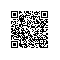# C语言例题23：

#include <stdio.h> void main() { int m,n,x,y; printf("输入两个正整数/n"); scanf("%d%d",&m,&n); if(m<n) //在m中存放m、n中最大者 { y=m; m=n; n=y; } printf("%d和%d的",m,n); y=m*n; //将m、n的值作个备份 while(x!=0) //辗转相除法 { x=m%n; m=n; n=x; } printf("最大公约数是%d/n最小公倍数是%d/n",m,y/m); }使用钉钉扫一扫加入圈子
+ 订阅